• 本文提出了基于支持向量拟合(Support vector regression,S V R)代理模型的优化策略,并将其应用于海洋卫星多学科设计优化中。本文算例卫星参考海洋一号卫星(HY-1),优化后整星质量相对于初始质量下降了约14.1E。优化...
• 支持向量机可以用来拟合线性回归。 相同的最大间隔（maximum margin）的概念应用到线性回归拟合。代替最大化分割两类目标是，最大化分割包含大部分的数据点（x，y）。我们将用相同的iris数据集，展示用刚才的概念来...
支持向量机可以用来拟合线性回归。  相同的最大间隔（maximum margin）的概念应用到线性回归拟合。代替最大化分割两类目标是，最大化分割包含大部分的数据点（x，y）。我们将用相同的iris数据集，展示用刚才的概念来进行花萼长度与花瓣宽度之间的线性拟合。
相关的损失函数类似于max（0，|yi-（Axi+b）|-ε）。ε这里，是间隔宽度的一半，这意味着如果一个数据点在该区域，则损失等于0。
# SVM Regression
#----------------------------------
#
# This function shows how to use TensorFlow to
# solve support vector regression. We are going
# to find the line that has the maximum margin
# which INCLUDES as many points as possible
#
# We will use the iris data, specifically:
#  y = Sepal Length
#  x = Pedal Width

import matplotlib.pyplot as plt
import numpy as np
import tensorflow as tf
from sklearn import datasets
from tensorflow.python.framework import ops
ops.reset_default_graph()

# Create graph
sess = tf.Session()

# iris.data = [(Sepal Length, Sepal Width, Petal Length, Petal Width)]
x_vals = np.array([x for x in iris.data])
y_vals = np.array([y for y in iris.data])

# Split data into train/test sets
train_indices = np.random.choice(len(x_vals), round(len(x_vals)*0.8), replace=False)
test_indices = np.array(list(set(range(len(x_vals))) - set(train_indices)))
x_vals_train = x_vals[train_indices]
x_vals_test = x_vals[test_indices]
y_vals_train = y_vals[train_indices]
y_vals_test = y_vals[test_indices]

# Declare batch size
batch_size = 50

# Initialize placeholders
x_data = tf.placeholder(shape=[None, 1], dtype=tf.float32)
y_target = tf.placeholder(shape=[None, 1], dtype=tf.float32)

# Create variables for linear regression
A = tf.Variable(tf.random_normal(shape=[1,1]))
b = tf.Variable(tf.random_normal(shape=[1,1]))

# Declare model operations

# Declare loss function
# = max(0, abs(target - predicted) + epsilon)
# 1/2 margin width parameter = epsilon
epsilon = tf.constant([0.5])
# Margin term in loss
loss = tf.reduce_mean(tf.maximum(0., tf.subtract(tf.abs(tf.subtract(model_output, y_target)), epsilon)))

# Declare optimizer
train_step = my_opt.minimize(loss)

# Initialize variables
init = tf.global_variables_initializer()
sess.run(init)

# Training loop
train_loss = []
test_loss = []
for i in range(200):
rand_index = np.random.choice(len(x_vals_train), size=batch_size)
rand_x = np.transpose([x_vals_train[rand_index]])
rand_y = np.transpose([y_vals_train[rand_index]])
sess.run(train_step, feed_dict={x_data: rand_x, y_target: rand_y})

temp_train_loss = sess.run(loss, feed_dict={x_data: np.transpose([x_vals_train]), y_target: np.transpose([y_vals_train])})
train_loss.append(temp_train_loss)

temp_test_loss = sess.run(loss, feed_dict={x_data: np.transpose([x_vals_test]), y_target: np.transpose([y_vals_test])})
test_loss.append(temp_test_loss)
if (i+1)%50==0:
print('-----------')
print('Generation: ' + str(i+1))
print('A = ' + str(sess.run(A)) + ' b = ' + str(sess.run(b)))
print('Train Loss = ' + str(temp_train_loss))
print('Test Loss = ' + str(temp_test_loss))

# Extract Coefficients
[[slope]] = sess.run(A)
[[y_intercept]] = sess.run(b)
[width] = sess.run(epsilon)

# Get best fit line
best_fit = []
best_fit_upper = []
best_fit_lower = []
for i in x_vals:
best_fit.append(slope*i+y_intercept)
best_fit_upper.append(slope*i+y_intercept+width)
best_fit_lower.append(slope*i+y_intercept-width)

# Plot fit with data
plt.plot(x_vals, y_vals, 'o', label='Data Points')
plt.plot(x_vals, best_fit, 'r-', label='SVM Regression Line', linewidth=3)
plt.plot(x_vals, best_fit_upper, 'r--', linewidth=2)
plt.plot(x_vals, best_fit_lower, 'r--', linewidth=2)
plt.ylim([0, 10])
plt.legend(loc='lower right')
plt.title('Sepal Length vs Pedal Width')
plt.xlabel('Pedal Width')
plt.ylabel('Sepal Length')
plt.show()

# Plot loss over time
plt.plot(train_loss, 'k-', label='Train Set Loss')
plt.plot(test_loss, 'r--', label='Test Set Loss')
plt.title('L2 Loss per Generation')
plt.xlabel('Generation')
plt.ylabel('L2 Loss')
plt.legend(loc='upper right')
plt.show()

输出结果：
-----------
Generation: 50
A = [[ 2.91328382]] b = [[ 1.18453276]]
Train Loss = 1.17104
Test Loss = 1.1143
-----------
Generation: 100
A = [[ 2.42788291]] b = [[ 2.3755331]]
Train Loss = 0.703519
Test Loss = 0.715295
-----------
Generation: 150
A = [[ 1.84078252]] b = [[ 3.40453291]]
Train Loss = 0.338596
Test Loss = 0.365562
-----------
Generation: 200
A = [[ 1.35343242]] b = [[ 4.14853334]]
Train Loss = 0.125198
Test Loss = 0.16121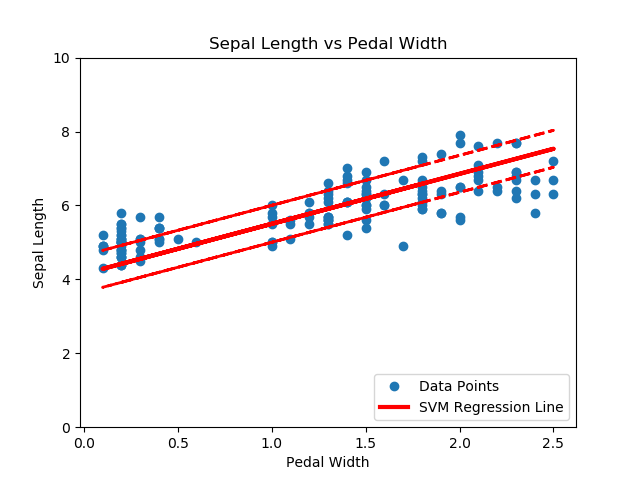基于iris数据集（花萼长度和花瓣宽度）的支持向量机回归，间隔宽度为0.5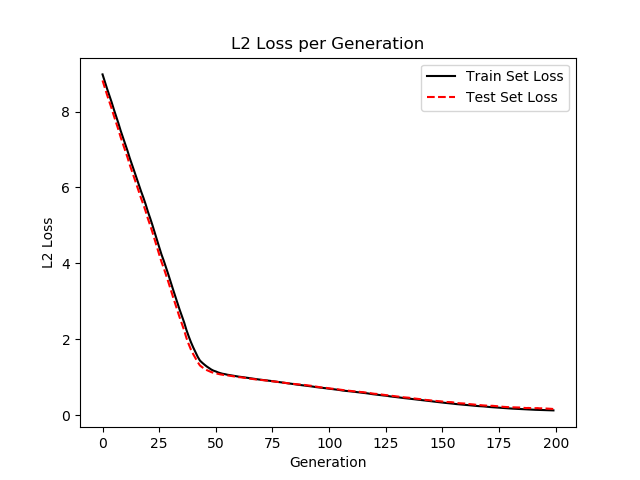每次迭代的支持向量机回归的损失值（训练集和测试集）
直观地讲，我们认为SVM回归算法试图把更多的数据点拟合到直线两边2ε宽度的间隔内。这时拟合的直线对于ε参数更有意义。如果选择太小的ε值，SVM回归算法在间隔宽度内不能拟合更多的数据点；如果选择太大的ε值，将有许多条直线能够在间隔宽度内拟合所有的数据点。作者更倾向于选取更小的ε值，因为在间隔宽度附近的数据点比远处的数据点贡献更少的损失。
展开全文• 在图像分析中，例如在 AFM 地形学领域，一个标准问题是将给定 ORDer 的多项式拟合到多列数据，被视为同一自变量的不同函数。 POLYFIC 以矢量化的方式（不使用循环）解决了这个... POLYVAC 可以使用生成的拟合向量矩阵matlab
•研究论文
• 一、创建向量  1、a=[1,2,3]  2、a=1:k:9 k为步长  3、a=linspace(1,10,k) k为向量中元素的个数 二、创建矩阵  1、a=[1,2,3;4,5,6]  2、a=reshape(1:12,3,[]) 3表示矩阵为3行  3、ones(3,4) 创建3行4列...
一、创建向量
1、a=[1,2,3]
2、a=1:k:9  k为步长
3、a=linspace(1,10,k)  k为向量中元素的个数
二、创建矩阵
1、a=[1,2,3;4,5,6]
2、a=reshape(1:12,3,[])  3表示矩阵为3行

3、ones(3,4)  创建3行4列矩阵  randi(10,[5,4])  创建5行4列 元素范围在1~10的矩阵
三、拟合一组散点

x=[2954.9,7866.9,5272.0];
y=[3281.5,8683.7,5354.6];
xi=1:1:8000;
p=polyfit(x,y,1)%  1表示1次拟合
yi=polyval(p,xi);
plot(x,y,'o',xi,yi)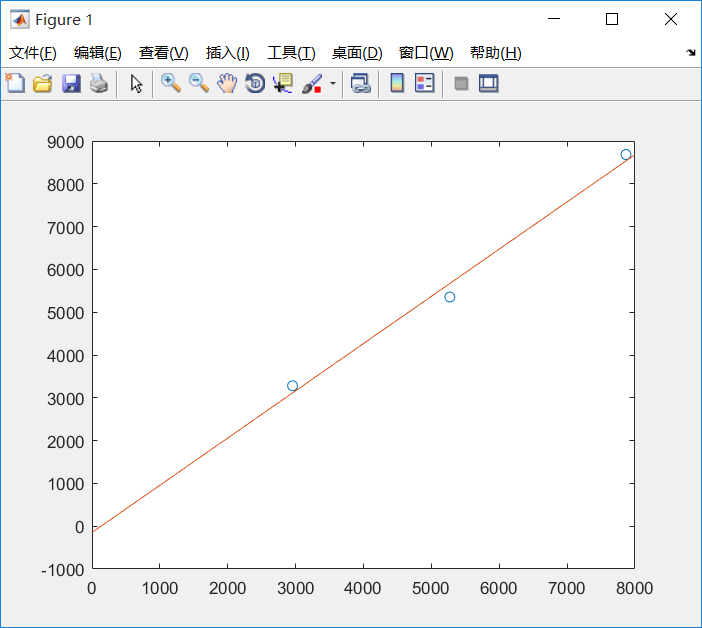展开全文• 支持向量机可以用来拟合线性回归。 相同的最大间隔（maximum margin）的概念应用到线性回归拟合。代替最大化分割两类目标是，最大化分割包含大部分的数据点（x，y）。我们将用相同的iris数据集，展示用刚才的概念...
• 神经网络仿真作业，设计支持向量机实现一对数组的函数拟合P=-1：0.1：1T=[-0.96 -0.577 ..... .....]使用支持向量机相应的回归函数svr， svrplot ， svroutput进行函数拟合的仿真实验中采用rbf核函数，惩罚因子取100...
• 点云法向量估计的主要思路是对K-近邻的N个点进行平面拟合（平面过N点重心），平面法向量即为所求；所以求法向量就是变相的求拟合平面。 下面我们用最小二乘法求k近邻点云的拟合平面： 当 ||x||＝1时，Ax=0的最小...
     点云法向量估计的主要思路是对K-近邻的N个点进行平面拟合（平面过N点重心），平面法向量即为所求；所以求法向量就是变相的求拟合平面。
下面我们用最小二乘法求k近邻点云的拟合平面：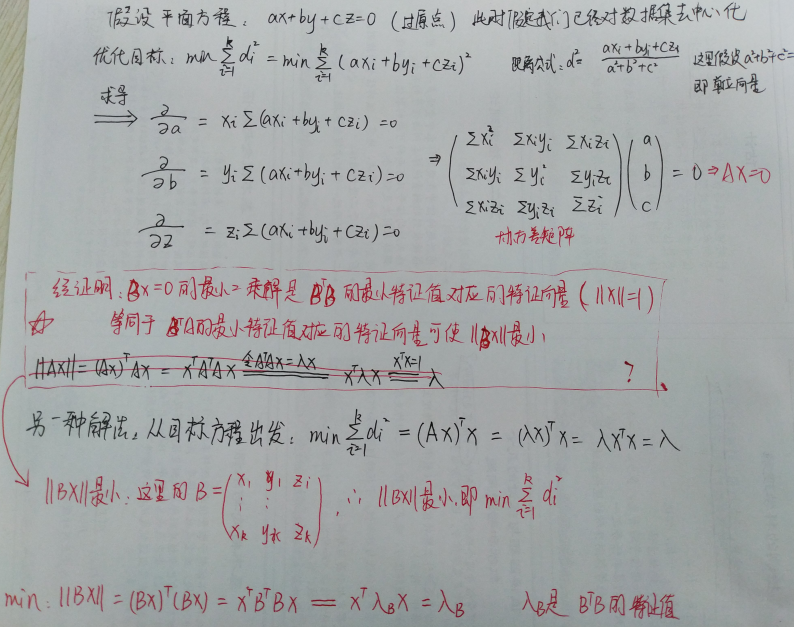当 ||x||＝1时，Ax=0的最小二乘解是ATA的最小特征值对应的特征向量等同于：ATA的最小特征值所对应的特征向量可使||Ax||最小。
结论：假设k-近邻点矩阵B，B为k*3的矩阵，则根据B拟合平面的法向量就是BTB对应的最小特征向量；
同理我们可以得出BTB对应的最大特征向量就是拟合平面的方向向量。（这里的前提是：B已经去中心化）

实际上，求一组点的拟合平面的过程，就是求其PCA的过程。因为PCA也是可以通过最小化投影距离推导出来的。即样本点到这个超平面的距离足够近。
PCA的推导:基于最小投影距离
重建：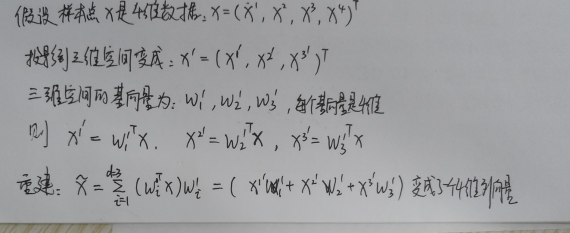上式中的x'其实表示原样本点在新的基下的拉伸程度系数，我们把拉伸系数再乘上对应的基向量（在原坐标系下的方向向量），就相当于求出投影后的样本点在原坐标系下的坐标。以三维坐标为例，将点P（x,y,z）投影到一个平面内，变成二维坐标M（x',y'）,平面使用2个基向量（基向量是3维）表示，（x',y'）再与2个基向量相乘求和，就得到点M在原三维空间的坐标。
即样本点到这个超平面的距离足够近。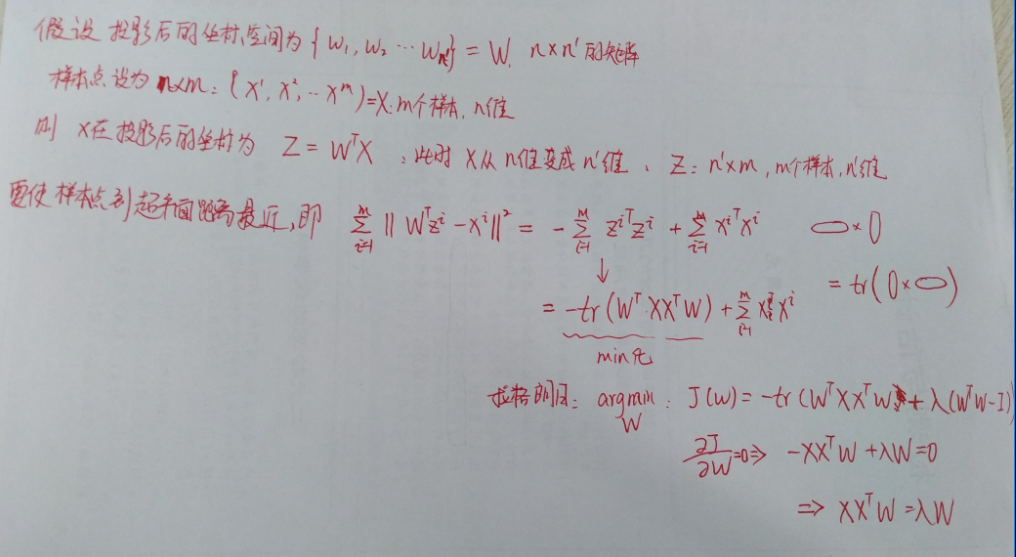展开全文• 拟合平面的方程为∏：a*x+b*y+c*z+d=0. 数据点Pi到平面a*x+b*y+c*z+d=0的距离设为di, 则di^2=(a*xi+b*yi+c*zi+d)^2/(a^2+b^2+c^2), 令L=∑di^2 （i=1,...,n）,为目标函数,现欲使L最小. L可以看成是关于(a,b,c,d)的...
转自：https://blog.csdn.net/z444_579/article/details/50039771
设有n个数据点Pi(xi,yi,zi).
假设平面方程为：a*x+b*y+c*z+d=0,其中a、b、c、d为待定系数a、b、c不能同时为0.

显然,a*x+b*y+c*z+d=0与

k*a*x+k*b*y+k*c*z+k*d=0（k≠0）

表示同一个平面.故,如d不为0,可通过把方程两边同除以d,把常数项化为1；但d=0时,情况稍微复杂一点.

现在说明大致思路,为讨论方便,开始时暂不假设d=1或0.

设拟合平面的方程为∏：a*x+b*y+c*z+d=0.

数据点Pi到平面a*x+b*y+c*z+d=0的距离设为di,

则di^2=(a*xi+b*yi+c*zi+d)^2/(a^2+b^2+c^2),

令L=∑di^2 （i=1,...,n）,为目标函数,现欲使L最小.

L可以看成是关于(a,b,c,d)的函数（(xi,yi,zi)均已知）,

L取最小值的一个必要（非充分）条件是：

∂L/∂a=0,∂L/∂b=0,∂L/∂c=0,∂L/∂d=0,

∂L/∂a=∑2*xi*(a*xi+b*yi+c*zi+d)/(a^2+b^2+c^2) （i=1,...,n）

=A1*a+B1*b+C1*c+D1*d,

其中,

A1=2/(a^2+b^2+c^2)*(∑xi^2)（i=1,...,n）,

B1=2/(a^2+b^2+c^2)*(∑xi*yi)（i=1,...,n）,

C1=2/(a^2+b^2+c^2)*(∑xi*zi)（i=1,...,n）,

D1=2/(a^2+b^2+c^2)*(∑xi)（i=1,...,n）,

同理,

∂L/∂b=A2*a+B2*b+C2*c+D2*d,

∂L/∂c=A3*a+B3*b+C3*c+D3*d,

其中,

A2=2/(a^2+b^2+c^2)*(∑yi*xi)（i=1,...,n）,

B2=2/(a^2+b^2+c^2)*(∑yi^2)（i=1,...,n）,

C2=2/(a^2+b^2+c^2)*(∑yi*zi)（i=1,...,n）,

D2=2/(a^2+b^2+c^2)*(∑yi)（i=1,...,n）,

A3=2/(a^2+b^2+c^2)*(∑zi*xi)（i=1,...,n）,

B3=2/(a^2+b^2+c^2)*(∑zi*yi)（i=1,...,n）,

C3=2/(a^2+b^2+c^2)*(∑zi^2)（i=1,...,n）,

D3=2/(a^2+b^2+c^2)*(∑zi)（i=1,...,n）,

∂L/∂d=∑2*(a*xi+b*yi+c*zi+d)/(a^2+b^2+c^2) （i=1,...,n）

=D1*a+D2*b+D3*c+D4*d,

其中,D4=2n/(a^2+b^2+c^2).

于是有方程组：

A1*a+B1*b+C1*c+D1*d=0,

A2*a+B2*b+C2*c+D2*d=0,

A3*a+B3*b+C3*c+D3*d=0,

D1*a+D2*b+D3*c+D4*d=0,

解此方程组即可.具体如何解,可参考计算方法的书,上面有详细说明.
千万注意：上述矩阵的秩rank<=3, 会的人立刻明白怎么结算了，不会的留言吧.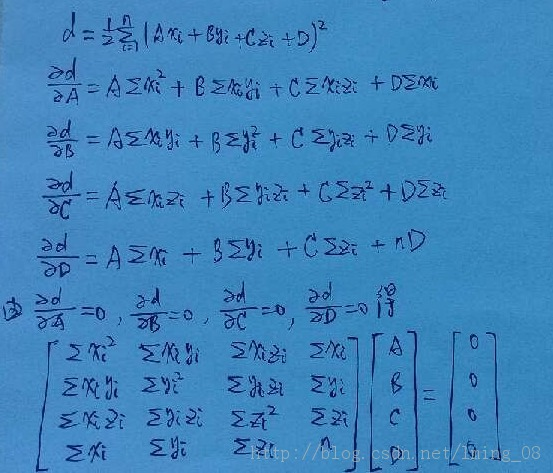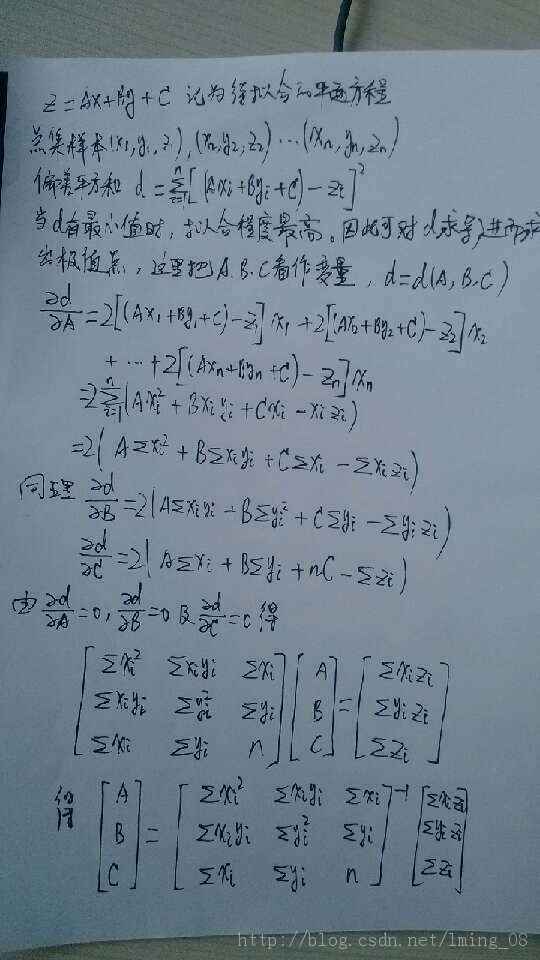展开全文最小二乘法 点云
• 论文，介绍支持向量机用于曲线拟合，基于支持向量机的散乱数据拟合
• 对于三维点云的每一个点利用邻域点拟合最小二乘平面并求得法向量
• 基于局部平面拟合求点云法向量，是我看到过的比较容易懂的，算法推导也写得很清楚点云处理
• 支持向量机的回归拟合——混凝土抗压强度预测，集合具体的案例给出了程序分析
• 支持向量拟合。很好用！ % Example usage: svrobj = svr_trainer(x_train,y_train,400,0.000000025,'gaussian',0.5); y = svrobj.predict(x_test);SVR
• chapter29 支持向量机的回归拟合——混凝土抗压强度预测
• 利用最小二乘支持做向量机回归预测分析，示例代码。
• 本节Jungle用C++实现最小二乘法拟合平面直线。 1.理论知识 平面直线的通用方程可以表示为 其中，A是直线的截距，B是直线的斜率。对于测量的二维坐标(x,y)，x是精确分布的，而y是观测值。基于最小二乘的理论，...最小二乘法
• 回归分析，函数估计的SVM，有三个参数控制着SVR的性能，包括平衡参数 、 ...如果 管道宽度过大，那么支持向量的数目越少，其拟合函数将不能反映真实的函数特性；反之，如果 管道宽度过小，那么SVR的稀疏性将不能保证。
• 代码解释的详细，可以直接用，已经测试过了，很好用。
• 三维曲线（非线性）拟合步骤 1 设定目标函数. （M函数书写）% 可以是任意的 例如： function f=mydata(a,data) %y的值目标函数值 或者是第三维的,a=[a(1) ，a(2)] 列向量 x=data(1,:); %data 是一2维数组，x=x1 y=...数学建模
• 空间直线最小二乘拟合理论推导如下：      从推导过程可以看出，只需求出四个参数即可确定一条直线，即求出矩阵即可。 ...
• 支持向量机的三要素： 模型：分离超平面 w*x+b=0 和决策函数 f(x)=sign(w*x+b) 策略：结构风险最小化，具体对于SVM是间隔最大化 算法：凸二次规划 对于支持向量机的模型和算法，都比较好理解，那它的策略间隔...SVM
• 可用于最小二乘支持向量回归相关问题，非线性拟合及预测
• 一、高维多元数据非线性/线性拟合： Matlab绘制三维空间网格散点图，使用cftool工具箱实现三维空间绘图。cftool工具箱是应用程序中的Curve Fitting应用。选择拟合曲线的类型，工具箱提供的拟合类型有： Custom ...数据分析
• 称呼： [系数，Rho，R2，残差] = linfit(x,y) 计算向量 'x' 和 'y' 之间的线性拟合，并返回回归系数线，'x' 和 'y' 之间的相关性，决定系数（“知道多少 x 它可以确定y“）和残差。如果残差表现出“非随机”模式，则...matlab
• 作者：Scofield ... 来源：知乎 著作权归作者所有。...很好，正好可借此机会介绍词向量、word2vec以及DeepNLP整套相关的东西： 文章很长，是从CSDN上写好复制过来的，亦可直接跳到博客观看： 深度学word2vec
• ## 最小二乘法曲线拟合以及Matlab实现

万次阅读 多人点赞 2017-12-28 17:31:23
最小二乘法曲线拟合以及Matlab实现 在实际工程中，我们常会遇到这种问题：已知一组点的横纵坐标，需要绘制出一条尽可能逼近这些点的曲线（或直线），以进行进一步进行加工或者分析两个变量之间的相互关系。而获取这...最小二乘法 matlab...